Example Questions

Example Question #31 : How To Find Order Of Operations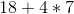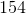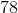Explanation:

There is multiplication and addition present. Remember PEMDAS. Multiplication comes first followed by addition.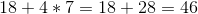Example Question #32 : How To Find Order Of Operations

When evaluating the expression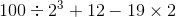what is the third operation that should be performed?

Cubing

Subtraction

Division

Multiplication

Multiplication

Explanation:

The order of operations is:

Exponents

Multiplication and division (left to right)

Addition and subtraction (left to right)

The first operation to be performed is the exponent (the cubing). The next two operations to be performed are the multiplication and the division; since the division is first when read left to right, this is performed second, and the multiplication is performed third.

Example Question #33 : How To Find Order Of Operations

Consider the expression: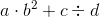.

Suppose you know the value of each of the four variables. In which order do you work the operations in the expression?

Explanation:

The order of operations is as follows:

Exponents

Multiplication and division (left to right)

Addition and subtraction (left to right)

The expression has an exponentiation - a squaring - so this is done first. It also has a multiplication and a division - the multiplication is to the left, so it is done next, followed by the division. This leaves the addition for last.

Example Question #34 : How To Find Order Of Operations

Consider the expression: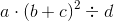.

Suppose you know the value of each of the four variables. In which order do you work the operations in the expression?

Explanation:

Any operation within parentheses is always done first - here, this is the addition.

Outside of this, the order of operations is as follows:

Exponents

Multiplication and division (left to right)

Addition and subtraction (left to right)

The expression has an exponentiation - a squaring - so this is done second. It also has a multiplication and a division - the multiplication is to the left, so it is done third, followed, finally, by the division.

Example Question #35 : How To Find Order Of Operations

Consider the expression: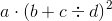.

Suppose you know the value of each of the four variables. In which order do you work the operations in the expression?

Explanation:

Any operation within parentheses is always done first. However, here, there are two operations within the parentheses, addition and division.

The order of operations is:

Exponents

Multiplication and division (left to right)

Addition and subtraction (left to right)

Therefore, the division is worked first, then the addition.

This leaves the exponentiation - here, a squaring - and a multiplication. By the above order, the squaring is worked third, then, finally, the multiplication.

Example Question #36 : How To Find Order Of Operations

When evaluating the expressionwhat is the last operation that should be performed?

Cubing

Multiplication

Division

Subtraction

Explanation:

The order of operations is:

Exponents

Multiplication and division (left to right)

Addition and subtraction (left to right)

Since there are no grouping symbols, and one of each operation, the addition and the subtraction will be the last two operations performed. When read left to right, the subtraction comes first, so the addition will be the last operation performed.

Example Question #37 : How To Find Order Of Operations

When evaluating the expression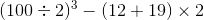what is the third operation that should be performed?

Subtraction

Multiplication

Cubing

Division

Cubing

Explanation:

The order of operations states that the expressions in the parentheses must be evaluated first. the first two operations performed will be the division and the addition. This leaves the cubing - an exponentiation - the subtraction, and the multiplication.

After grouping symbols, the order of operations is:

Exponents

Multiplication and division (left to right)

Addition and subtraction (left to right)

The cubing will be performed next - and third overall.

Example Question #38 : How To Find Order Of Operations

Solve: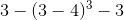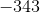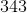Explanation:

To evaluate this expression, first simplify the quantity inside the parentheses and the exponent by order of operations.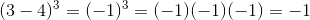Rewrite the expression.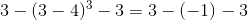Simplify the double negative.  It will turn into a positive sign.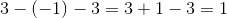The answer is:Example Question #39 : How To Find Order Of Operations

Solve: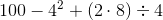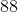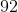Explanation:

Following the order of operations, we can simplify this expression.

Recall the order of operations:

PEMDAS

Parentheses, Exponents, Multiplication/Division, Addition/Subtraction. Perform these operations from left to right.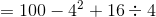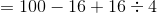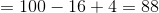Example Question #40 : How To Find Order Of Operations

Evaluate the expression and solve for x: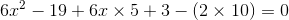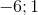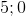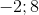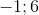Explanation:

In order to find the solutions for x, you first need to simplify the equation and properly use the order of operations. The order of operations is PEMDAS- Parentheses, Exponents, Multiplication/Division, Addition/Subtraction.

In this equation:you have to do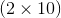first which equalsSecondly,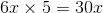You are left with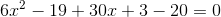, this simplifies to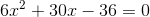After you have simplified the equation, you can factor the equation.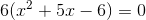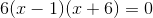Therefore the solutions are